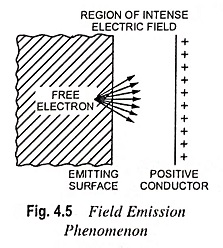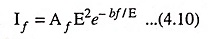## Field Emission or Cold Cathode Emission:

The metal piece can also be made to emit electrons by creating an high intensity electrostatic field near it. Such an emission of electrons is known as field emission or cold cathode emission.

If an electrode (anode) having sufficiently high positive potential with respect to the metal piece (cathode) is brought near it (cathode), it (anode) will attract the electrons with such a force that electrons will emit from the surface of the emitter (Fig. 4.5).This type of emission is almost independent of temperature and is a function of electric field intensity. The relation between field emission current and electric field intensity is given by the following equationwhere

• If is the field emissions current density
• E is the electric field intensity at the emitter surface
• Af is an approximately constant coefficient
• bf is a constant determined by the work function of the metal and
• e is the natural logarithmic base.

Very intense electric field (usually, a voltage of the order of a million of volts per cm distance between the emitting surface and the positive conductor) is required to produce field emissions. It can be had at temperatures much lower (e.g. room temperature) than required for thermionic emission and, therefore, it is also sometimes called cold cathode emission.

Scroll to Top